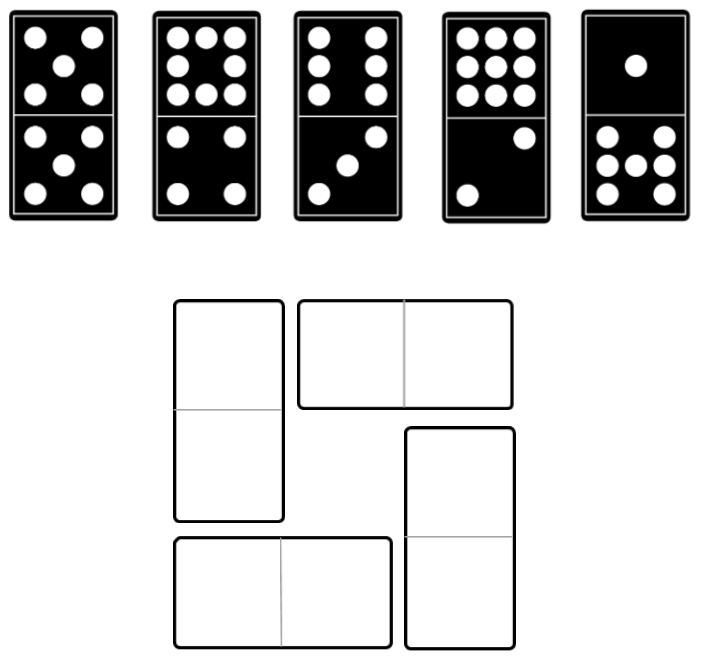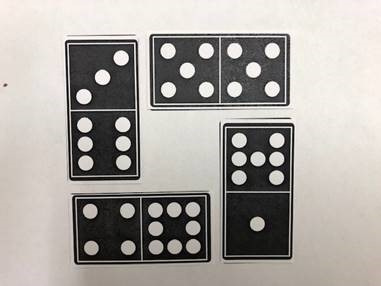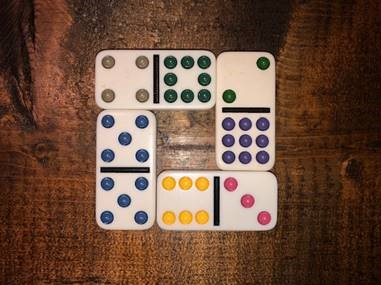Home > Grade 1 > Domino Window

# Domino Window

Directions: Use four of these dominoes to form a square with the same number of dots on each side.### Hint

What is the largest sum you can make?
What is the smallest sum you can make?

There are two known answers, one where the sum or each side is equal to 13.Here’s another where the sum of each side is equal to 14.Source: Joshua Nelson

### Check Also

Directions: Use the digits 1-9, at most once, to complete the equation. What is the …

1.We solved the domino puzzle and got a sum of 14 on each side. We don’t think 11 or 12 are possible. Can you explain?

•My first grade student also solved each side for the total of 14.

•You are correct, 11 and 12 are not possible. But those are the possible solutions to the other Domino Window task if your students what to give it a try! Thanks for bringing this to my attention!

•im in 4th grade and i got confused

2.You are correct, 11 and 12 are not possible. But those are the possible solutions to the other Domino Window task if your students want to give it a try! Thanks for bringing this to my attention!

3.4.5.I came up with 13 and 14.